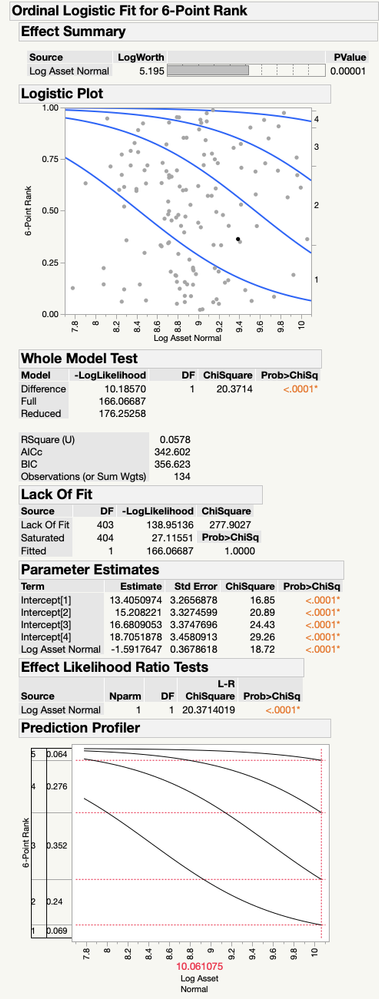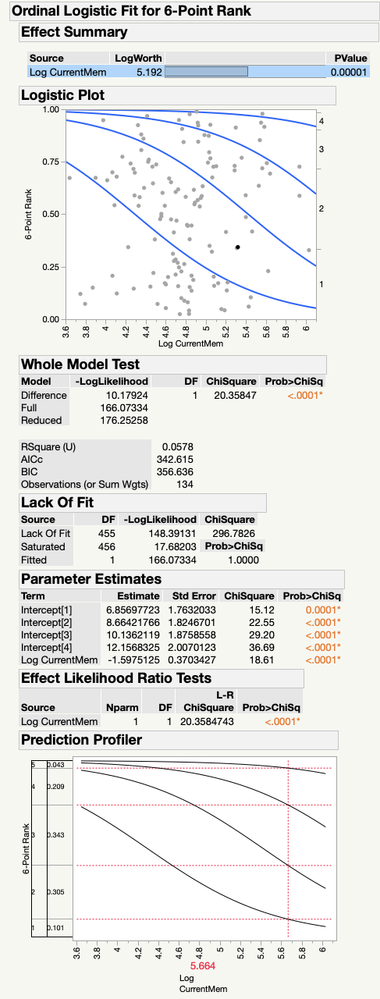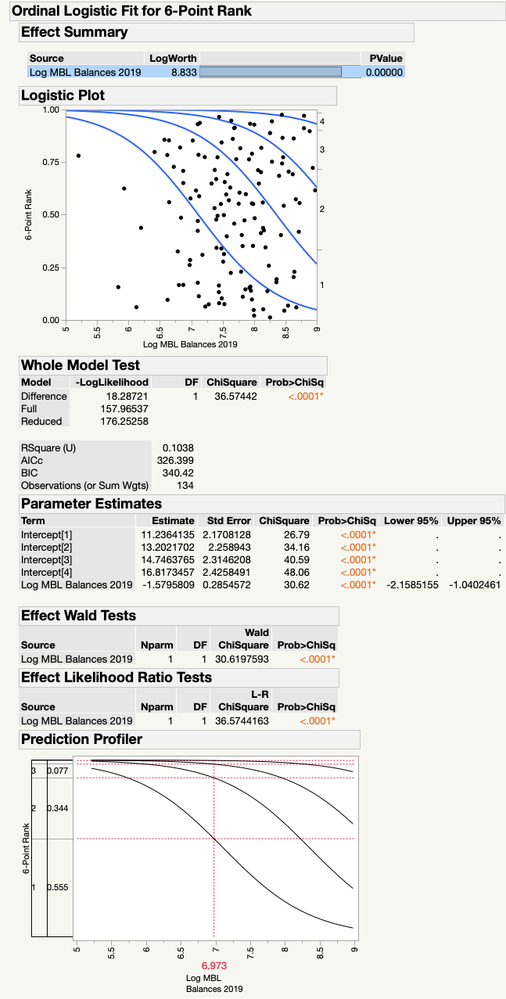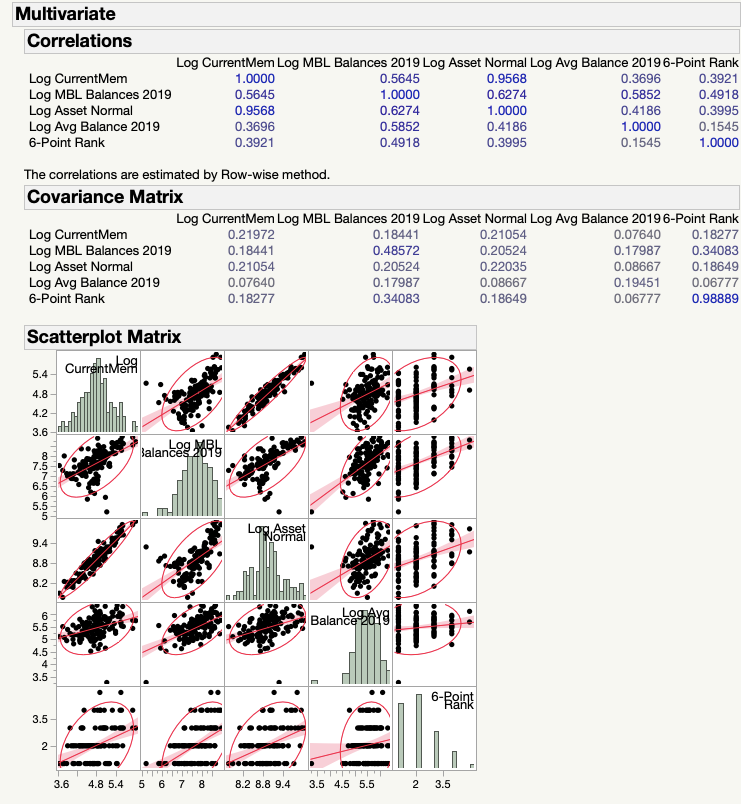Our World Statistics Day conversations have been a great reminder of how much statistics can inform our lives. Do you have an example of how statistics has made a difference in your life? Share your story with the Community!
Choose Language Hide Translation Bar
Highlighted

## How to determine greatest effect for ordinal logistic regressions

Hello,

I am testing the effects of four variables on a 6-point index using separate ordinal logistic regressions. Three of my variables show significant results but I am having trouble assessing which of these has the greatest effect on the probability of achieving higher ranks on my index.

I am posting my effect summaries for reference. My variable (LOG MBL Balances) exhibits the highest log-worth and highest pseudo r-squared but a slightly lower coefficient estimate than the other two variables. My other two variables (LOG assets and LOG membership) exhibit very similar results and both have a higher absolute value for avg log-likelihood than MBL balances.

Am I able to say that since LOG MBL exhibits a better fit that it would be the variable with the greatest effect on my DV despite having a lower coefficient and a lower absolute value on the avg log-likelihood? Or is it better to say that all three variables exhibit similar, modest effects on my DV?P.S. I have run my regressions in both JMP 14 and Eviews 11. Can anyone tell me why the coefficients are reported as negative in JMP while in Eviews they are reported as positive?

Thank you

1 ACCEPTED SOLUTION

Accepted Solutions
Highlighted

## Re: How to determine greatest effect for ordinal logistic regressions

The PCA is a very helpful tool in multivariate analysis of real data. Correlations are difficult to avoid. Hopefully the PCA alone provides some insight, such as assets and membership possibly the expression of some latent variable.

I assume that by "although factor 3 provides the lowest explanatory value (at ~12%)" you mean 12% of the variance in the PCA. You are correct about factor 3. The fact that factor 1 represents the most variation in the original variables in no way implies that it is the most important predictor. Using all three factors in the model provides a strong analysis for separating and testing the effects.

Learn it once, use it forever!
11 REPLIES 11
Highlighted

## Re: How to determine greatest effect for ordinal logistic regressions

There might be confusion about practical significance (effect size) and statistical significance (small p-value). I would use AICc to select a model, and then use information about the selected model to assess predictor.

You are also at a higher risk of a higher type I error by testing the predictors individually. There are only a few predictors in this case but in another situation there might be many.

Did you try to regress a model with all the predictors instead of individual models?

Are these predictors associated with each other? It is interesting that they produce such similar regression results.

You have a sparse level 3 and very sparse level 4, so be careful with interpretation.

I am not familiar with Eviews and I do not support it, but perhaps another community member can answer this question. You can see from the mosaic plots that the logistic curve is sloped downward, hence the negative coefficient for the predictor.

Learn it once, use it forever!
Highlighted

## Re: How to determine greatest effect for ordinal logistic regressions

I understand each of my three variables to be statistically significant and that they appear to have a modest practical effect on my DV. Does the AICc refer mainly to practical significance in this case? Will the larger AICc refer to a larger practical significance?

Yes, the variables indeed seem to be associated with each other. I am testing them individually because they each exhibit high multicollinearity with each other, hence when I try them together hence my results are very distorted.

Could you speak a little more to what it means that the logistic curve is downward sloping? Is this the same as saying that unit changes in my IV decrease the odds of ending up in level 1 (and thus higher odds of ending up in higher levels)?

Highlighted

## Re: How to determine greatest effect for ordinal logistic regressions

We cannot use information criteria, such as AICc, to assess significance. They are more of a probabilistic statement. The smaller the AICc, then the larger the likelihood of the parameters, given the fixed data, with a penalty for model complexity. The penalty is the same for all of your candidate models, so the difference is entirely the likelihood.

If they are highly correlated, then they are redundant. (No independent information) Can you pick one from prior theory or knowledge?

Are the predictor levels set or measured?

The logistic curves in the plot show a decrease in the probability of each of the ordinal levels as the predictor levels increase. The slope is negative. The ordinal logistic regression uses cumulative logits. The odds are not about one level versus another but sums of logits versus other sums. You could use the generalized logit in a nominal logistic regression analysis if that form of odds is more meaningful or useful.

You could try adding  square of the IV to the model and compare AICc to decide if there is an improvement. The response (logit) might not be linear.

Learn it once, use it forever!
Highlighted

## Re: How to determine greatest effect for ordinal logistic regressions

My research goal is to assess the respective effects of each of these variables. If the results are redundant, is there any other way I can do this, another kind of model perhaps? Or am I forced to really only pick one?

With a negative sloping logistic curve am I able to say that the magnitude of the effect is in the desired direction (that increases in my IV are associated with increased probabilities of higher levels?).

Highlighted

## Re: How to determine greatest effect for ordinal logistic regressions

How were the IV selected for the study? How were the levels determined? Were they set (e.g., factor levels) or measured (e.g., covariates)?

What is the correlation in the IV? Can you show the Multivariate platform with the matrix of correlations and the scatter plots?

The issue is that if the correlations are high, then this data set cannot assess the effects of the IV independently. The data cannot be used to say which one had the effect.

Yes, the negative slope means that the probability shifts from the lowest level to the highest level as the predictor level increases.

Learn it once, use it forever!
Highlighted

## Re: How to determine greatest effect for ordinal logistic regressions

I am testing the effect of factors related to scale on the adoption of non-lending business services among credit unions. My IV each represent some aspect of institutional scale (asset size, total membership, lending portfolio, average member balance). The levels here are measured.

The levels for my DV are constructed as an index using information about the provision of business services from each credit union; higher levels reflect more advanced kinds of business services.

Here is my multivariate matrixHighlighted

## Re: How to determine greatest effect for ordinal logistic regressions

Thank you for sharing those details. That information is helpful.

There are some strong correlations but not so strong as to call these IV redundant. So putting them together in the same model caused a lot of problems? They are collinear as you said but that should only inflate the variance. Was the problem with significance? You might try a PCA and then rotate the largest components to save as factors. They might be interpretable when you examine the loading and they might serve as surrogate IV while avoiding the collinearity problem.

Learn it once, use it forever!
Highlighted

## Re: How to determine greatest effect for ordinal logistic regressions

Yes, it was particularly bad whenever the asset size and current membership variables were together. It would mess with the significance of the model and change the sign of some coefficients. I could have a workable model if I only tested three variable (leaving out either assets or membership) but I felt as if testing them separately would be more appropriate for my research aims in general.

Do you think the series of models I've posted are validly interpretable as it is? (at least with respect to levels 1-3)

Thanks for the tip regarding PCA, I will look into that. Would you happen to have some quick link or tutorial handy for doing what you mentioned with rotating the largest components from PCA for use as surrogate IV through JMP? No worries if not, this was very helpful!

Highlighted

## Re: How to determine greatest effect for ordinal logistic regressions

I did not mean to question the validity of the analysis. Validity depends on the study design and the selection of the variables. I was only cautioning against the practice sometimes referred to as "p-value phishing." I am not suggesting that you are phishing. I think that you have demonstrated a good study. I just want to clarify my concern.

Let me illustrate with an extreme example. A fictional and aggressive study gathers hundreds of variables based on availability and tests each one against a response. Many variables (large number, not proportion) are concluded to have a significant effect. All that result means is that a pattern of correlation or association was discovered. There are many questions that cannot be answered by the data that was used to obtain that result.

• Is that result simply a matter of type I error due to the large number of hypothesis tests?
• Are the significant variables reasonable based on current theory?
• Are the results another example of spurious correlations?

New data must be collected to verify and strengthen the belief in the initial findings. Valid studies and findings are reproducible. If we skip the verification and rush to report it, then it is probably a case of phishing.

So a valid study is a planned exploration, starting with a theory, that considers the appropriate data collection and methods beforehand. Designed experiments are very successful studies, albeit not always possible. A valid study must try to obtain empirical evidence for and against the theory. We hope that there is no evidence against our theory, but we are still obliged to look for it. We don't want someone else to find it for us.

Learn it once, use it forever!
Article Labels

There are no labels assigned to this post.